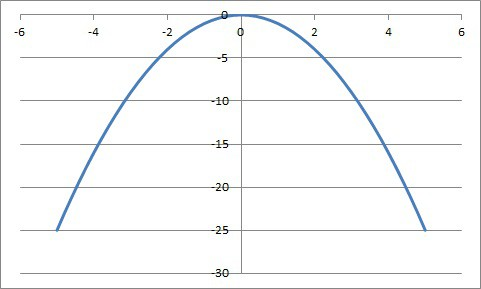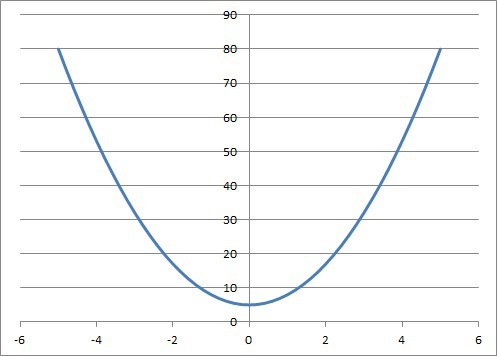# What Do The Solutions Of A Quadratic Equation Represent?

You will spend a lot of time in algebra classes trying to find the solutions of quadratic equations.  However, knowing how to find these solutions is not the same as knowing what they mean.

So, what do the solutions of a quadratic equation represent?  The solutions of a quadratic equation represent x values where the equation is true.  We can factor a quadratic equation in standard form with these solutions.  Visually, the solutions of a quadratic equation represent the points where a parabola intersects the x-axis (that is, where y = 0).

Of course, for a quadratic equation in standard form, the solutions tell us when the left side is equal to zero.  For a quadratic function f(x), the solutions of the quadratic equation tell us when f(x) = 0.

In this article, we’ll talk about the solutions of a quadratic equation and what they represent.  We’ll also take a closer look at some of the cases for the solutions and when they occur.

Let’s get started.

## What Do The Solutions Of A Quadratic Equation Represent?

The solutions of a quadratic equation represent the values of x that make the equation true.  For a quadratic equation in standard form, these x values will make the left side of the equation equal to zero.

For a quadratic function f(x), the solutions are the values of x that make y equal to zero.  These x values are also called the roots of the quadratic equation.

That is, if x = a is a solution to the quadratic function f(x), then f(a) = 0.

For example, consider the quadratic function f(x) = x2 – 1, with solutions are x = 1 and x = -1.  We can see this if we factor it as a difference of squares:

• f(x) = x2 – 1
• f(x) = (x + 1)(x – 1)  [factor as a difference of squares]

We can verify these two solutions by substituting them for x in the quadratic function:

• f(1) = 12 – 1 = 1 – 1 = 0
• f(-1) = (-1)2 – 1 = 1 – 1 = 0

The solutions of a quadratic equation also represent a way for us to factor.  Given the quadratic function f(x) = ax2 + bx + c, the solutions x = r and x = s give us the complete factorization:

• f(x) = a(x – r)(x – s)

For example, consider the quadratic function f(x) = 5x2 -15x + 10.  If we know that the roots are x = 1 and x = 2, then we can factor completely with a = 5, r = 1, and s = 2:

• f(x) = a(x – r)(x – s)
• f(x) = 5(x – 1)(x – 2)

We can use FOIL and the Distributive Property to verify this:

• f(x) = 5(x – 1)(x – 2)
• f(x) = 5(x2 – 2x – x + 2)
• f(x) = 5(x2 – 3x + 2)
• f(x) = 5x2 – 15x + 10

Of course, the solutions of a quadratic equation also have a visual interpretation.  They represent very specific points on the graph of a parabola.

### What Do The Solutions Of A Quadratic Equation Represent On A Graph?

The solutions of a quadratic equation represent the points on a graph where a parabola intersects the x-axis.  That is, when y = 0 or when f(x) = 0.

Remember that we can graph a quadratic function f(x) = ax2 + bx + c as a parabola (shown below).

Depending on the solutions, a parabola may intersect the x-axis twice, once, or not at all.  The number of intersections is determined by the type of solutions: two distinct real roots, one repeated real root, or two complex conjugate roots.

Remember that a parabola is a function, but it is not a one-to-one function. So, it can intersect the x-axis (the line y = 0) more than once.

To determine the exact solutions a quadratic equation has, we can use the quadratic formula (shown below).

After putting the quadratic equation into standard form, ax2 + bx + c = 0, we can find a, b, and c to use in the quadratic formula.

To find the nature of the solutions of a quadratic equation (what type of solutions we have), we can use one part of the formula: the discriminant.

The discriminant of a quadratic equation is the expression under the radical symbol in the quadratic formula: b2 – 4ac.

There are three cases for the sign of the discriminant, corresponding to the type of solutions for a quadratic equation:

• Positive Discriminant: when b2 – 4ac > 0, we have two distinct real solutions to the quadratic equation.
• Zero Discriminant: when b2 – 4ac = 0, we have one repeated real solution to the quadratic equation.
• Negative Discriminant: when b2 – 4ac < 0, we have two complex conjugate solutions to the quadratic equation.

The table and graphic below shows the various cases for solutions of a quadratic equation and what the corresponding graph would have for x-axis intersections.

The solutions of a quadratic equation are significant because they allow us to factor a quadratic equation in standard form (as long as we know the value of a, the coefficient of x2 in the equation).

The solutions of a quadratic in standard form also tell us where the corresponding parabola will intersect the x-axis (if at all) when we graph the function.  This makes it easier to draw the graph, since we can use the solutions to help us.

## What Is The Greatest Number Of Solutions That A Quadratic Equation Can Have?

The greatest number of real solutions that a quadratic equation can have is two.  In fact, a quadratic equation has exactly two complex solutions, although they may not always be real numbers.

As we learned earlier, a quadratic equation can have two distinct real solutions, one repeated real solution, or two complex conjugate solutions.

The limit of two solutions for a quadratic equation is guaranteed by the Fundamental Theorem of Algebra, which states that:

• A polynomial of degree n in one variable with complex coefficients has n complex roots.

Since a quadratic equation has degree n = 2, we know that it has exactly two solutions.  The repeated real root (when the discriminant is zero) counts as two solutions, due to multiplicity.The vertex of this parabola rests on the x-axis, meaning that it represents a quadratic equation with one real repeated root (here, a double root of x = 0).

Knowing what a parabola looks like (always the same concavity and one turning point) makes it easy to see that a quadratic equation can have at most two real solutions.  These solutions represent the points (if any) where the parabola intersects the x axis (y = 0).

### How Many Real Solutions Can A Quadratic Equation Have?

A quadratic equation can have 0, 1, or 2 real solutions.  This depends entirely on the sign of the discriminant, b2 – 4ac (the expression under the radical in the quadratic formula):

• Positive Discriminant: when b2 – 4ac > 0, a quadratic equation has two real solutions.
• Zero Discriminant: when b2 – 4ac = 0, a quadratic equation has one real solution (a double root).
• Negative Discriminant: when b2 – 4ac > 0, a quadratic equation has no real solutions (instead, it has two complex conjugate solutions).

Note that the cases where the discriminant is nonzero (positive or negative) are the cases where we have a quadratic equation with two solutions.

The table below illustrates the different cases for real solutions of a quadratic, depending on the sign of the discriminant.

### How Can A Quadratic Equation Have No Solution?

A quadratic equation will always have solutions, but they may not always be real.  For a quadratic equation to have no real solution, we must have two complex conjugate solutions.

This happens when the discriminant is negative (b2– 4ac < 0).

When we graph such a quadratic equation, the resulting parabola will not intersect the x-axis at all.  That is, the value of y will never be zero, as you can see below:This parabola represents the graph of a quadratic equation with no real solutions (it has two complex conjugate solutions). Note that it never intersects the x-axis.

The parabola will either:

• Always be above the x-axis (y > 0 for all x), which can happen when a > 0 and c > 0.
• Always be below the x-axis (y < 0 for all x), which can happen when a < 0 and c < 0.

Note that we can only get complex solutions with a nonzero imaginary part for a quadratic equation when a and c have the same sign (both positive or both negative).

## Conclusion

Now you know exactly what the solutions of a quadratic equation represent, both algebraically and graphically.  You also know when the various cases (two real, one real, or two complex conjugate solutions) occur.